# Kantorovich process

An iterative method for improving the approximation to the value of a root of a non-linear functional (operator) equation (a generalization of Newton's method cf. Newton method). For the equation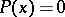, whereis a non-linear operator from one Banach space to another, the formula for calculating the root has the form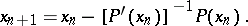(Here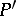is the Fréchet derivative.) Sometimes a modified process, given by the following formula, is used: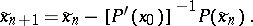Suppose that the operatoris twice continuously differentiable and that the following conditions hold (see ):

1) the linear operator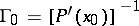exists;

2);

3)when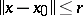;

4)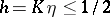;

5).

Then the equationhas a solution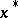such that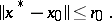The sequences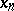and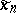converge to this solution, with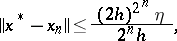and in the case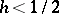,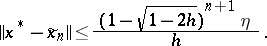The Kantorovich process always converges to a rootof the equation, provided thatis sufficiently smooth,exists and the initial approximationis chosen sufficiently close to. Ifexists and is continuous, then the convergence of the basic process is quadratic. The rate of convergence of the modified process is that of a decreasing geometric progression; the denominator of this progression tends to zero as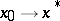.

The process was proposed by L.V. Kantorovich .